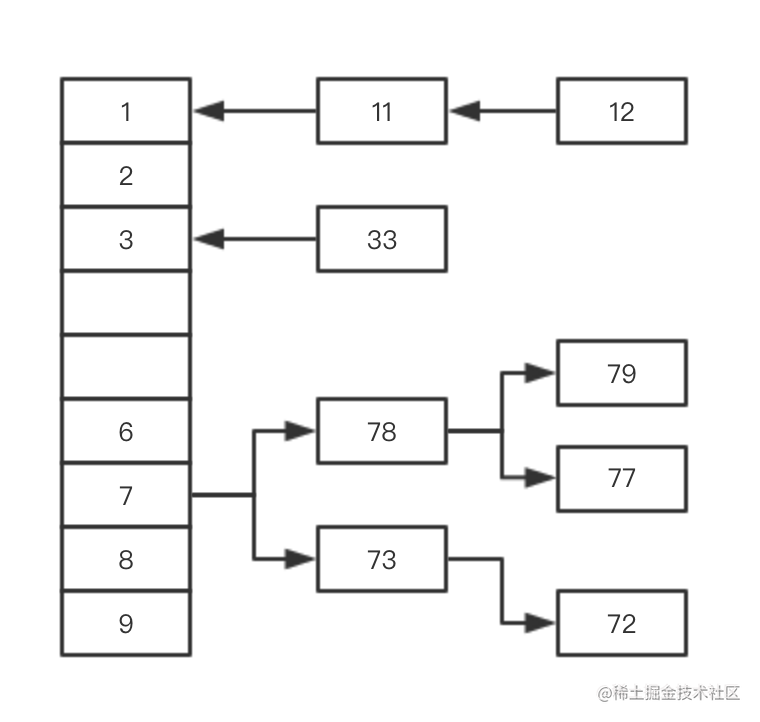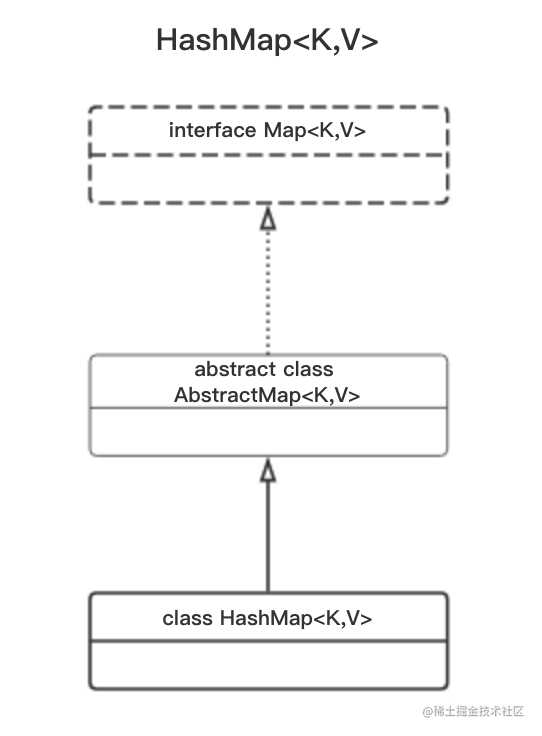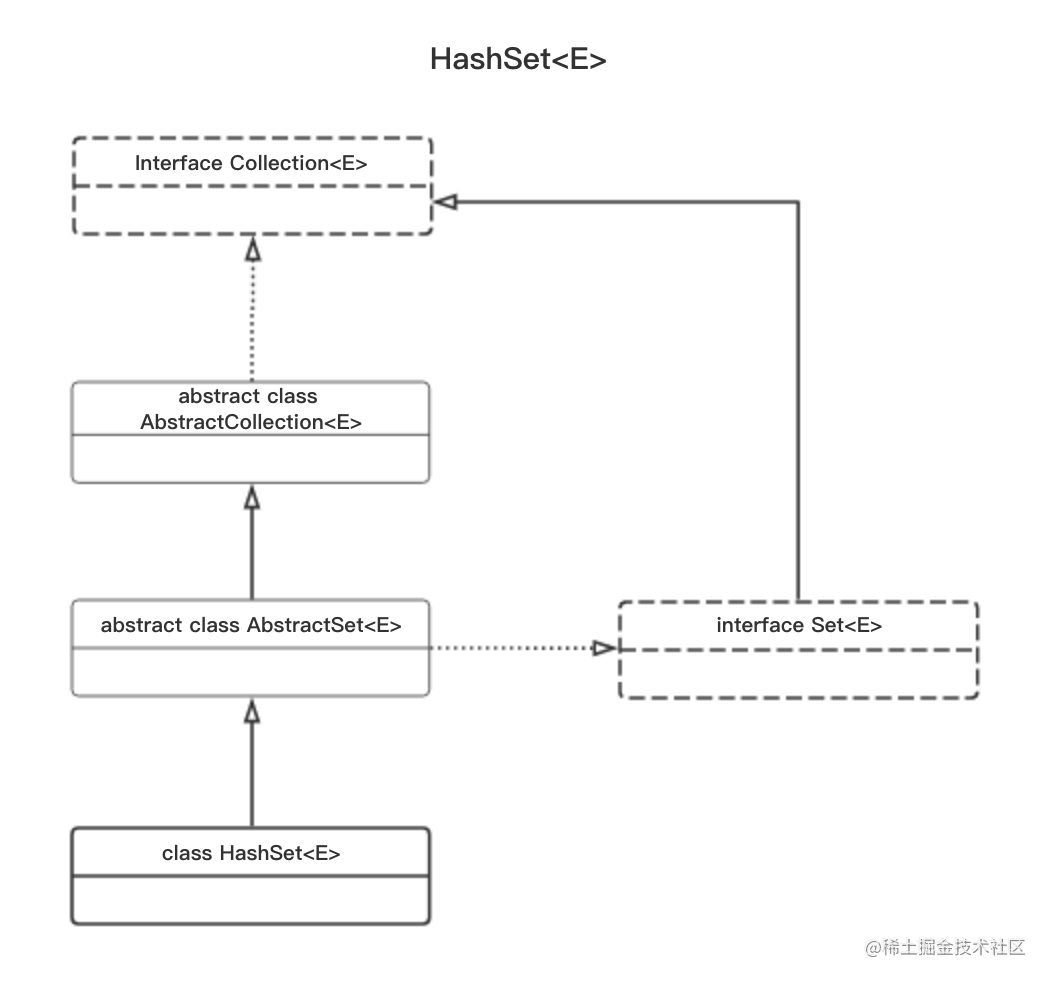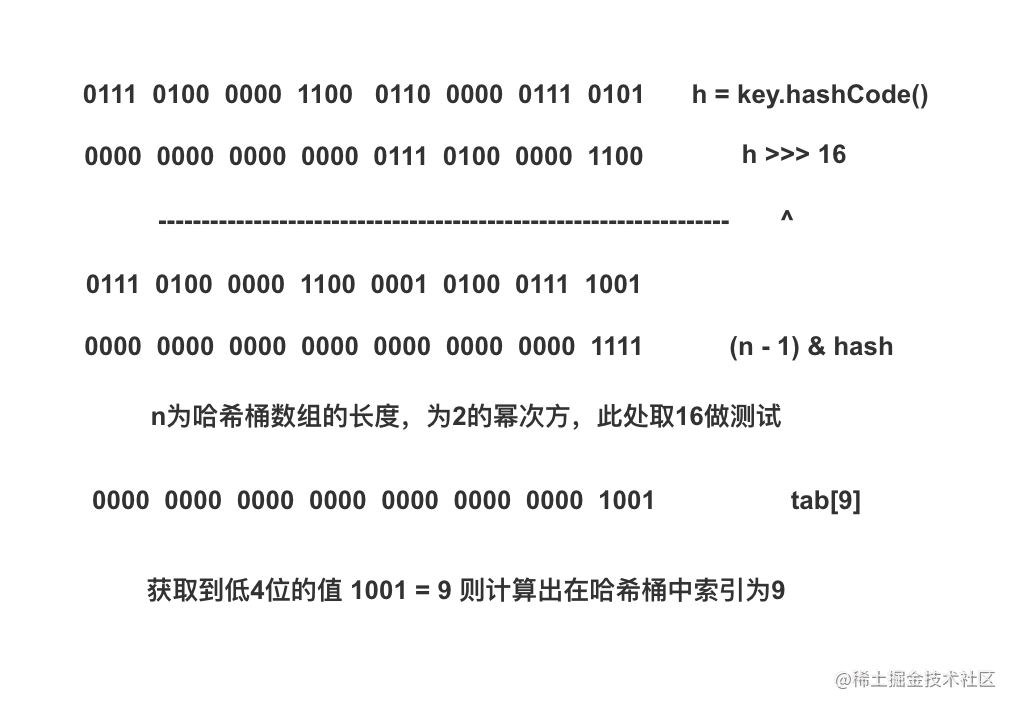# Java集合（5）一 HashMap与HashSet

## 引言

HashMap<K,V>和TreeMap<K,V>都是从键映射到值的一组对象，不同的是，HashMap<K,V>是无序的，而TreeMap<K,V>是有序的，相应的他们在数据结构上区别也很大。

HashMap<K,V>在键的数据结构上采用了数组，而值在数据结构上采用了链表或红黑树这两种数据结构。HashSet<K,V>同HashMap<K,V>的关系与TreeSet<E>同TreeMap<K,V>的关系类似，在内部实现上也是使用了HashMap<K,V>的键集，这点我们同样通过HashSet<K,V>的构造函数可以发现。所以在文章中只会详细解说HashMap<K,V>，对HashSet<K,V>就不做分析。

``````public HashSet() {
map = new HashMap<>();
}

public HashSet(int initialCapacity) {
map = new HashMap<>(initialCapacity);
}

## 框架结构

HashMap<K,V>在继承结构上和TreeMap<K,V>类似，都继承自AbstractMap<K,V>，同时也都实现了Map<K,V>接口，所以在功能上区别不大，不同的是实现功能的底层数据结构。同时由于HashMap<K,V>是无序的，没有继承自SortedMap<K,V>，相应的少了一些根据顺序查找的功能。## 构造函数

``````public class HashMap<K,V> extends AbstractMap<K,V> implements Map<K,V>, Cloneable, Serializable  {
//默认填充因子
static final float DEFAULT_LOAD_FACTOR = 0.75f;
//哈希桶数组
transient Node<K,V>[] table;

public HashMap(int initialCapacity, float loadFactor) {
if (initialCapacity < 0)
throw new IllegalArgumentException("Illegal initial capacity: " + initialCapacity);
if (initialCapacity > MAXIMUM_CAPACITY)
initialCapacity = MAXIMUM_CAPACITY;
//临界值（第一次临界值为转换后的容量大小）
this.threshold = tableSizeFor(initialCapacity);
}

//默认填充因子 threshold（第一次临界值为转换后的容量大小）
public HashMap(int initialCapacity) {
}

//默认填充因子 threshold临界值为0
public HashMap() {
}
}

``````static final int tableSizeFor(int cap) {
//避免出现输入8变成16这种情况
int n = cap - 1;
n |= n >>> 1;
n |= n >>> 2;
n |= n >>> 4;
n |= n >>> 8;
n |= n >>> 16;
//低位全变为1之后，进行n + 1可以将低位全变为0，得到2的幂次方
return (n < 0) ? 1 : (n >= MAXIMUM_CAPACITY) ? MAXIMUM_CAPACITY : n + 1;
}

## 哈希值

``````static final int hash(Object key) {
int h;
return (key == null) ? 0 : (h = key.hashCode()) ^ (h >>> 16);
}使用2的整数次幂可以很方便的通过tab[(n - 1) & hash]获取到哈希桶所需要的低位值，由于低位和高位进行了异或运算，保留了高低位的特征，也就减少了哈希值冲突的可能性。这就是为什么这里会使用2的整数次幂来初始化哈希桶数组长度的原因。

## 添加元素

``````public V put(K key, V value) {
return putVal(hash(key), key, value, false, true);
}

final V putVal(int hash, K key, V value, boolean onlyIfAbsent, boolean evict) {
Node<K,V>[] tab; Node<K,V> p; int n, i;
//哈希桶数组为空时，通过resize初始化哈希桶数组
if ((tab = table) == null || (n = tab.length) == 0)
n = (tab = resize()).length;
//哈希值所对应的位置为空，代表不会产生冲突，直接赋值即可
if ((p = tab[i = (n - 1) & hash]) == null)
tab[i] = newNode(hash, key, value, null);
else {
//产生哈希冲突
Node<K,V> e; K k;
//如果哈希值相等，并且key也相等，则直接覆盖值
if (p.hash == hash && ((k = p.key) == key || (key != null && key.equals(k))))
e = p;
//p为红黑树 使用红黑树逻辑进行添加（可以查看TreeMap）
else if (p instanceof TreeNode)
e = ((TreeNode<K,V>)p).putTreeVal(this, tab, hash, key, value);
//p为链表
else {
for (int binCount = 0; ; ++binCount) {
//查找到链表末尾未发现相等元素则直接添加到末尾
if ((e = p.next) == null) {
p.next = newNode(hash, key, value, null);
//链表长度大于8时，扩容哈希桶数组或将链表转换成红黑树
if (binCount >= TREEIFY_THRESHOLD - 1) // -1 for 1st
treeifyBin(tab, hash);
break;
}
//遍历链表过程中存在相等元素则直接覆盖value
if (e.hash == hash && ((k = e.key) == key || (key != null && key.equals(k))))
break;
p = e;
}
}
//覆盖value
if (e != null) { // existing mapping for key
V oldValue = e.value;
if (!onlyIfAbsent || oldValue == null)
e.value = value;
afterNodeAccess(e);
return oldValue;
}
}
++modCount;
//哈希桶中的数据大于临界值时扩容
if (++size > threshold)
resize();
afterNodeInsertion(evict);
return null;
}

final void treeifyBin(Node<K,V>[] tab, int hash) {
int n, index; Node<K,V> e;
//哈希桶数组小于64则扩容哈希桶数组
if (tab == null || (n = tab.length) < MIN_TREEIFY_CAPACITY)
resize();
else if ((e = tab[index = (n - 1) & hash]) != null) {
TreeNode<K,V> hd = null, tl = null;
//将所有Node<K,V>节点类型的链表转换成TreeNode<K,V>节点类型的链表
do {
TreeNode<K,V> p = replacementTreeNode(e, null);
if (tl == null)
hd = p;
else {
p.prev = tl;
tl.next = p;
}
tl = p;
} while ((e = e.next) != null);
//将TreeNode<K,V>链表转换成红黑树
if ((tab[index] = hd) != null)
hd.treeify(tab);
}
}

## 扩容

``````final Node<K,V>[] resize() {
Node<K,V>[] oldTab = table;
int oldCap = (oldTab == null) ? 0 : oldTab.length;
int oldThr = threshold;
int newCap, newThr = 0;
//哈希桶数组已经初始化 则直接向左位移1位 相当于扩容一倍
if (oldCap > 0) {
if (oldCap >= MAXIMUM_CAPACITY) {
threshold = Integer.MAX_VALUE;
return oldTab;
}
//向左位移1位 扩容一倍
else if ((newCap = oldCap << 1) < MAXIMUM_CAPACITY && oldCap >= DEFAULT_INITIAL_CAPACITY)
newThr = oldThr << 1; // double threshold
}
//哈希桶数组未初始化 并且已经初始化容量
else if (oldThr > 0) // initial capacity was placed in threshold
newCap = oldThr;
//哈希桶数组未初始化 并且未初始化容量 则使用默认容量DEFAULT_INITIAL_CAPACITY
else {               // zero initial threshold signifies using defaults
newCap = DEFAULT_INITIAL_CAPACITY;
}
//初始化哈希桶数组容量以及临界值
if (newThr == 0) {
float ft = (float)newCap * loadFactor;
newThr = (newCap < MAXIMUM_CAPACITY && ft < (float)MAXIMUM_CAPACITY ?
(int)ft : Integer.MAX_VALUE);
}
threshold = newThr;
@SuppressWarnings({"rawtypes","unchecked"})
//初始化哈希桶数组
Node<K,V>[] newTab = (Node<K,V>[])new Node[newCap];
table = newTab;
//将所有元素拷贝到新哈希桶数组中
if (oldTab != null) {
for (int j = 0; j < oldCap; ++j) {
Node<K,V> e;
if ((e = oldTab[j]) != null) {
oldTab[j] = null;
//不存在哈希冲突，重新计算哈希值并拷贝
if (e.next == null)
newTab[e.hash & (newCap - 1)] = e;
//冲突结构为红黑树
else if (e instanceof TreeNode)
((TreeNode<K,V>)e).split(this, newTab, j, oldCap);
//冲突结构为链表
else { // preserve order
Node<K,V> loHead = null, loTail = null;
Node<K,V> hiHead = null, hiTail = null;
Node<K,V> next;
do {
next = e.next;
//扩容后最高位为0，则不需要移动到新的位置
if ((e.hash & oldCap) == 0) {
if (loTail == null)
else
loTail.next = e;
loTail = e;
}
//扩容后最高位为1，则需要移动
else {
if (hiTail == null)
else
hiTail.next = e;
hiTail = e;
}
} while ((e = next) != null);
if (loTail != null) {
loTail.next = null;
}
//对哈希值改变的节点移动到新的位置
if (hiTail != null) {
hiTail.next = null;
}
}
}
}
}
return newTab;
}

## 查找元素

``````public V get(Object key) {
Node<K,V> e;
//计算哈希值
return (e = getNode(hash(key), key)) == null ? null : e.value;
}

final Node<K,V> getNode(int hash, Object key) {
Node<K,V>[] tab; Node<K,V> first, e; int n; K k;
if ((tab = table) != null && (n = tab.length) > 0 &&
(first = tab[(n - 1) & hash]) != null) {
//哈希值相等，并且key也相等，则返回查找到的值
if (first.hash == hash && // always check first node
((k = first.key) == key || (key != null && key.equals(k))))
return first;
//哈希值存在冲突，第一个不是要找的key
if ((e = first.next) != null) {
//冲突结构为红黑树
if (first instanceof TreeNode)
return ((TreeNode<K,V>)first).getTreeNode(hash, key);
//冲突结构为链表
do {
if (e.hash == hash &&
((k = e.key) == key || (key != null && key.equals(k))))
return e;
} while ((e = e.next) != null);
}
}
return null;
}

## 删除元素

``````public V remove(Object key) {
Node<K,V> e;
//计算哈希值
return (e = removeNode(hash(key), key, null, false, true)) == null ?
null : e.value;
}

final Node<K,V> removeNode(int hash, Object key, Object value,
boolean matchValue, boolean movable) {
Node<K,V>[] tab; Node<K,V> p; int n, index;
if ((tab = table) != null && (n = tab.length) > 0 &&
(p = tab[index = (n - 1) & hash]) != null) {
Node<K,V> node = null, e; K k; V v;
//哈希值相等，并且key也相等，则node查找到的节点
if (p.hash == hash &&
((k = p.key) == key || (key != null && key.equals(k))))
node = p;
//哈希值存在冲突，第一个不是要找的key
else if ((e = p.next) != null) {
//冲突结构为红黑树
if (p instanceof TreeNode)
node = ((TreeNode<K,V>)p).getTreeNode(hash, key);
//冲突结构为链表
else {
do {
if (e.hash == hash &&
((k = e.key) == key ||
(key != null && key.equals(k)))) {
node = e;
break;
}
p = e;
} while ((e = e.next) != null);
}
}
if (node != null && (!matchValue || (v = node.value) == value ||
(value != null && value.equals(v)))) {
//节点为红黑树节点，按红黑树逻辑删除
if (node instanceof TreeNode)
((TreeNode<K,V>)node).removeTreeNode(this, tab, movable);
//节点为桶中第一个节点
else if (node == p)
tab[index] = node.next;
//节点为后续节点
else
p.next = node.next;
++modCount;
--size;
afterNodeRemoval(node);
return node;
}
}
return null;
}